Courses

# Phase Relations of Soils Civil Engineering (CE) Notes | EduRev

## Civil Engineering (CE) : Phase Relations of Soils Civil Engineering (CE) Notes | EduRev

The document Phase Relations of Soils Civil Engineering (CE) Notes | EduRev is a part of the Civil Engineering (CE) Course Soil Mechanics.
All you need of Civil Engineering (CE) at this link: Civil Engineering (CE)

Phase Relations of Soils

Soil is not a coherent solid material like steel and concrete, but is a particulate material. Soils, as they exist in nature, consist of solid particles (mineral grains, rock fragments) with water and air in the voids between the particles. The water and air contents are readily changed by changes in ambient conditions and location.

As the relative proportions of the three phases vary in any soil deposit, it is useful to consider a soil model which will represent these phases distinctly and properly quantify the amount of each phase. A schematic diagram of the three-phase system is shown in terms of weight and volume symbols respectively for soil solids, water, and air. The weight of air can be neglected.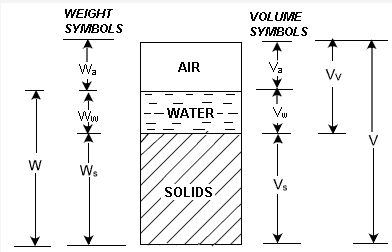The soil model is given dimensional values for the solid, water and air components.

Total volume, V = Vs + Vw + Vv

Three-phase System

Soils can be partially saturated (with both air and water present), or be fully saturated (no air content) or be perfectly dry (no water content).

In a saturated soil or a dry soil, the three-phase system thus reduces to two phases only, as shown.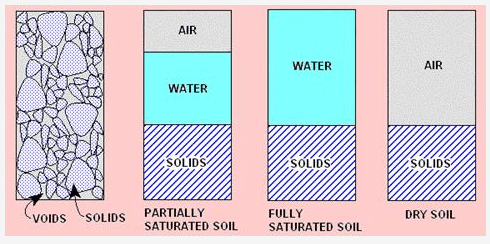For the purpose of engineering analysis and design, it is necessary to express relations between the weights and the volumes of the three phases.

The various relations can be grouped into:

• Volume relations
• Weight relations
• Inter-relations

Volume Relations

As the amounts of both water and air are variable, the volume of solids is taken as the reference quantity. Thus, several relational volumetric quantities may be defined. The following are the basic volume relations:

1. Void ratio (e) is the ratio of the volume of voids (Vv) to the volume of soil solids (Vs), and is expressed as a decimal.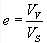2. Porosity (n) is the ratio of the volume of voids to the total volume of soil (V ), and is expressed as a percentage.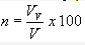Void ratio and porosity are inter-related to each other as follows: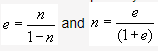3. The volume of water (Vw) in a soil can vary between zero (i.e. a dry soil) and the volume of voids. This can be expressed as the degree of saturation (S) in percentage.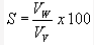4. Air content (ac) is the ratio of the volume of air (Va) to the volume of voids.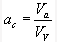5. Percentage air voids (nais the ratio of the volume of air to the total volume.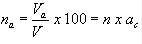Offer running on EduRev: Apply code STAYHOME200 to get INR 200 off on our premium plan EduRev Infinity!

## Soil Mechanics

31 videos|48 docs|42 tests

,

,

,

,

,

,

,

,

,

,

,

,

,

,

,

,

,

,

,

,

,

;